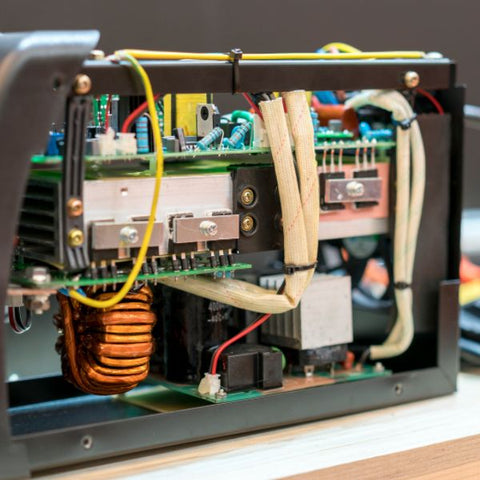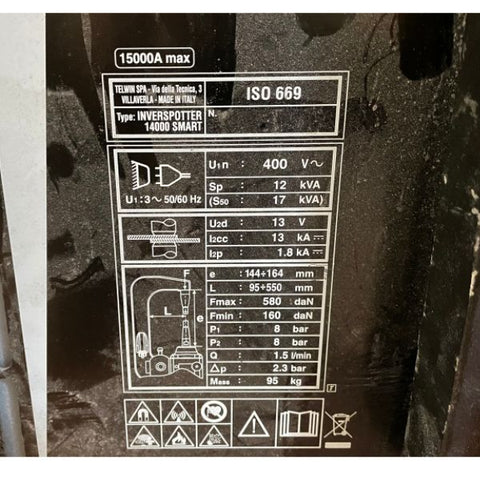WE SHIP WORLDWIDE - PRICES EXCLUDE TAX -ORDERS ABOVE £100 FREE CARRIAGE - WE SHIP WORLDWIDE
WE SHIP WORLDWIDE - PRICES EXCLUDE TAX

# What Size Generator Do I Need?

This is one of the most common questions we get asked and its important especially when it comes to Inverter welders and possible site work what size generator will I need?.

When hiring or using a generator you will often be asked "what size Do you Need?" Generator sizes are measured in kilovolt-amperes (kVA) and its important that you get the correct size to run your equipment as damage to both your inverter and your generator can occur if you select wrongly.WHAT IS KVA?

kVA is the "Apparent" power or how much power you can work with.

WHAT IS KWA?

Kilowatt is the "Actual" power a system has ie the actual usable output.

If kW is how much power you can work with, kVA tells you how much is being used.

Generators are always measured in kVA

POWER FACTOR

If an electrical circuit was perfectly efficient  then a kW would be equal to a kVA. But because no system is completely efficient, not all of the apparent power will be converted into useful output. There will be losses. We therefore need to take these losses into consideration when working out.  This is what we call "power factor" and in general generators have a power factor of 0.8CONVERTING KVA TO KWA

To convert kW to kVA, you first need to know the efficiency (or power factor) of the system. Power factor (pf) is measured on a scale from 0 to 1.

On average, a generator will have a power factor of 0.8.

 kW to kVA is kW / Power factor kVA to kW kVA x PF

Example kW to kVA:

100kW divided by power factor 0.8 = 80kVA

Example kVA to kW:

100kVA times  Power Factor 0.8 = 80kWWHAT IS VOLT DROP?

This is the important part in this and the reason its critical in selecting a generator large enough to run your equipment.

Inverters are a parallel system if you ask for a lot of current out (welding) then it will require it to draw more current in (supply) to feed this.

If the current you ask for in welding cannot be supplied fast enough by the supply then you will experience a volts drop in the circuit and this in turn means more current could flow causing current (amps) to rise even further until either the supply trips or the internals of the inverter blow.

This is why this fluctuation is dangerous to inverters, these peaks and troughs of you arcing up and down causes voltage fluctuations in the inverter and this can prove fatal if its allowed too much.

Ive tried to use inverter spot welders before on an inverter and actually managed to nearly stop the generator from turning due to the load I put on it. This I found quite scary and I wouldn't recommend you try it.LOOK AT THE SPECIFICATION PLATE

Some but not all manufacturers put the kVA required on the specification plate.

The example above is for a spot welder which we normally wouldn't recommend use on a generator, but as you can see its a 400v welder and at 50% power (S50) it requires a 17kVA generator.WHAT IF IT DOESN'T SHOW kVA?

We need to do some more maths to work this one out. to work out the "watts" we need to use:

Watts= Volts x Amps.

230x25= 5750

or 5.7kW

Convert to kVA

kW ÷ Pf

5750 ÷ 0.8 = 11.5KVA

You would need an 11.5 kVA generator to run this welder at full power.

kW to kVA CONVERSION CHART

 kW kVA 5 6.3 7.5 9.3 10 12.5 15 18.720 20 25 25 31.3